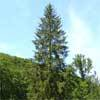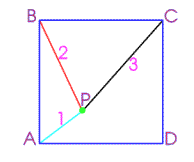#### You may also like### Baby Circle

A small circle fits between two touching circles so that all three circles touch each other and have a common tangent? What is the exact radius of the smallest circle?### Logosquares

Ten squares form regular rings either with adjacent or opposite vertices touching. Calculate the inner and outer radii of the rings that surround the squares.### Two Trees

Two trees 20 metres and 30 metres long, lean across a passageway between two vertical walls. They cross at a point 8 metres above the ground. What is the distance between the foot of the trees?

# Square World

##### Age 16 to 18Challenge Level

 $P$ is a point inside a square $ABCD$ such that $PA= 1$, $PB = 2$ and $PC = 3$. How big is ${\angle APB}$ ?